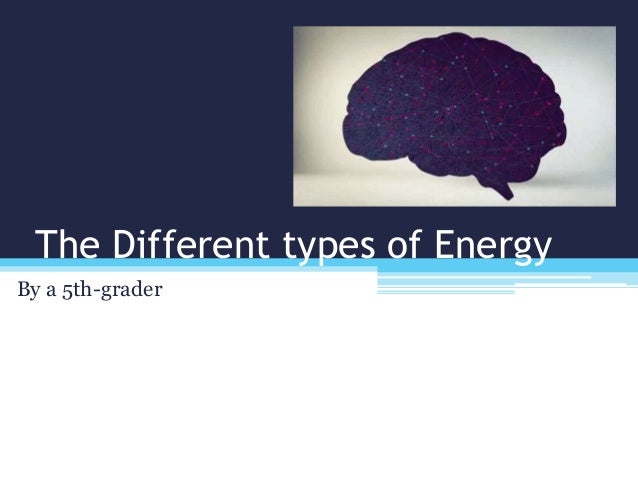Successfully reported this slideshow.Upcoming SlideShare
×

# The Different Types of Energy

A slideshow report from a 5th-grader
Slide 1: Title
Slide 2: What is energy
Slide 3: Electrical energy
Slide 4: Heat energy
Slide 5: Light energy
Slide 6: Chemical energy
Slide 7: Sound energy
Slide 8: Nuclear energy
Slide 9: Mechanical energy
Slide 10: Kinetic energy
Slide 11: Potential energy
Slide 12: End

• Full Name
Comment goes here.

Are you sure you want to Yes No### The Different Types of Energy

1. 1. The Different types of Energy By a 5th-grader
2. 2. What is energy? Energy is the ability to do work. Energy can change an objects motion, temperature, colour, shape.
3. 3. Electrical energy Electric energy is the energy created by electrons moving through an electrical conductor.
4. 4. Heat energy Heat energy is the total of kinetic and potential energy of the atoms in an object.
5. 5. Light energy Light energy is the only form of energy that we can actually see directly. Light energy can also be converted into other forms of energy.
6. 6. Chemical energy • Chemical Energy is energy stored in the bonds of chemical compounds that are atoms and molecules. • It forms new substances like sound, light, thermal.
7. 7. Sound energy It is a form of energy associated with the vibration o
8. 8. It is the energy obtained by manipulating the inte by dividing the nucleus or by the joining of two a Nuclearenergy
9. 9. Mechanical energy It is the sum of kinetic energy and potential en
10. 10. Kinetic energy Kinetic energy is the energy of motion. An o
11. 11. Potential energy It is energy an object has because of its posit
12. 12. Thank you!